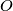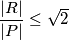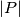### IMO Shortlist 2009 problem G5

Kvaliteta:
Avg: 0,0
Težina:
Avg: 8,0
Let$P$ be a polygon that is convex and symmetric to some point$O$. Prove that for some parallelogram$R$ satisfying$P\subset R$ we havewhere$|R|$ and$|P|$ denote the area of the sets$R$ and$P$, respectively.

Proposed by Witold Szczechla, Poland
Izvor: Međunarodna matematička olimpijada, shortlist 2009